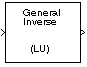# LU Inverse

Compute inverse of square matrix using LU factorization

• Library:
• DSP System Toolbox / Math Functions / Matrices and Linear Algebra / Matrix Inverses

•## Description

The LU Inverse block computes the inverse of the square input matrix A by factoring and inverting its row-pivoted variant Ap.

`${A}_{p}^{-1}={\left(LU\right)}^{-1}$`

L is a lower triangular square matrix with unity diagonal elements, and U is an upper triangular square matrix. The block outputs the inverse matrix A-1.

## Ports

### Input

expand all

Specify the input as an M-by-M square matrix.

Data Types: `single` | `double`
Complex Number Support: Yes

### Output

expand all

Matrix inverse, returned as an M-by-M square matrix.

Data Types: `single` | `double`
Complex Number Support: Yes

## Block Characteristics

 Data Types `double` | `single` Direct Feedthrough `no` Multidimensional Signals `no` Variable-Size Signals `no` Zero-Crossing Detection `no`

 Golub, Gene H., and Charles F. Van Loan. Matrix Computations. 3rd ed. Baltimore, MD: Johns Hopkins University Press, 1996.